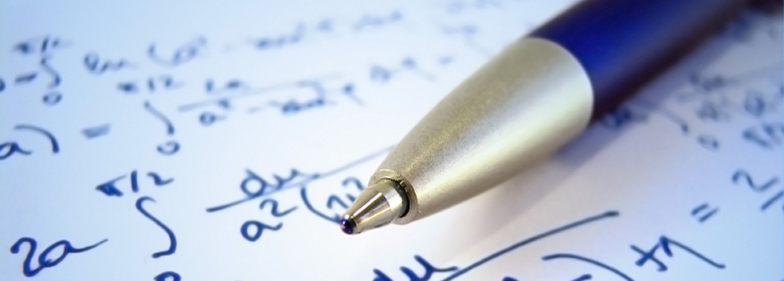﻿ Calculus 12 Tutoring | Math Tutor Network Vancouver

## CALCULUS 12 / AP CALCULUS 12 TUTORING### Overview

Calculus 12 are intended for students who would like to get a head start to university math. Students shall have fundamental knowledge of algebra, graphing, functions, and trigonometry. Students enrolled in AP Calculus 12 and obtained an adequate score on the exam may obtain university credits.

### Key Concepts

• Limits and Continuity
• Derivative and Differentiation Techniques (product, quotient, chain rule, implicit differentiation)
• Applications of Derivative (related rates, extrema, optimization, linear approximations)
• Curve Sketching (higher derivatives, asymptotes, concavity)
• Integration by Riemann sums
• Integration by parts
• Applications of Integration
• Polar Differentiation and Polar Coordinates

### Common Difficulties

The most common problem students have in Calculus is in tackling application word problems for both derivatives and integration. Utilizing information given into the formulas may be challenging and requires a lot of practice to be proficient at it. Students shall have a solid understanding of each theory taught (i.e.: what the formula calculates vs. knowing how to use the formula). Analytic Geometry involving planar curves of polar and vector functions is another area students often struggle with.

### How Math Tutor Network can help?

Math Tutor Network has specialized calculus tutors. Tutors may be TA's from university who have previously teaching experience in first-year calculus, experts in the field, or teachers with a solid calculus background.

### Our Recommendations

With or without tutoring lessons, students should always complete their homework/assignment as best as they can. A good approach is to give each question the best effort before checking the answers. Before quizzes or tests, try to do a set of questions and resist from checking the answer until all questions are completed. By doing so, students will be more prepared in a test-writing environment.

### Tutoring Lessons

For students who only require help on homework problems and for test preparation, Math Tutor Network recommends one 2-hour lesson per week.

For students having difficulties understanding the concepts the teacher go over in class, Math Tutor Network recommends two or more 2-hour lessons per week.